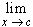#Interactive Real Analysis

Next | Previous | Glossary | Map

## 6.1. Limits

### Proposition 6.1.7: Properties for limits of Functions

Iff(x) exists, the limit is unique.[ f(x) + g(x) ] =f(x) +g(x), provided thatf(x) andg(x) exist.[ f(x) g(x) ] =f(x)g(x), provided thatf(x) andg(x) exist.[ f(x) / g(x) ] =f(x) /g(x), provided thatf(x) andg(x) exist andg(x) # 0.

### Proof:

Next | Previous | Glossary | Map